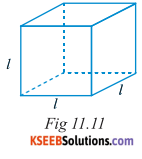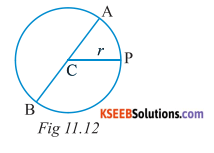# KSEEB Solutions for Class 6 Maths Chapter 11 Algebra Ex 11.2

Students can Download Chapter 11 Algebra Ex 11.2 Questions and Answers, Notes Pdf, KSEEB Solutions for Class 6 Maths helps you to revise the complete Karnataka State Board Syllabus and score more marks in your examinations.

## Karnataka State Syllabus Class 6 Maths Chapter 11 Algebra Ex 11.2

Question 1.
The side of an equilateral triangle is shown by l. Express the perimeter of the equilateral triangle using l
Solution:
Side of equilateral ∆ le = l
Perimeter = l + l + l + 3l

Question 2.
The side of a regular hexagon(Fig 11.10) is denoted by l. Express the perimeter of the hexagon using l.
(Hint: A regular hexagon has all its six sides equal in length)Sides of regular hexagon = l
Perimeter = 6lQuestion 3.
A cube is a three – dimensional figure as shown in Fig 11.11. It has six faces and all of them are identical squares. The length of an edge of the cube is given by l. Find the formula for the total length of the edges of a cube.
Solution:
length of edge = l
Number of edges = 12
Total length of the edges = Number of edges × length of one edge = 12lQuestion 4.
The diameter of a circle is a line which joins two points on the circle and also passes through the centre of the circle. (In the adjoining figure (Fig 11.12 )AB is a diameter of the circle ; C is its centre). Express the diameter of the circle (d) in terms of its radius(r).
Solution:Diameter = AB = AC + CB = r + r = 2r
d = 2rQuestion 5.
To find sum of three numbers 14, 27 and 13, we can have two ways:
a) We may first add 14 and 27 to get 41 and then add 13 to it to get the total sum 54 or
b) We may add 27 and 13 to get 40 and then add 14 to get 40 and then add 14 to get the sum 54. Thus, (14 + 27) + 13 = 14 + (27 + 13)
This can be done for any three numbers, his property is known as the associativity of addition of numbers. Express this property which we have already studied in the chapter on whole numbers, in a general way, by using variables a, b and c.
Solution:
For any three whole numbers a, b, and c
(a + b) + c = a + (b + c)

error: Content is protected !!